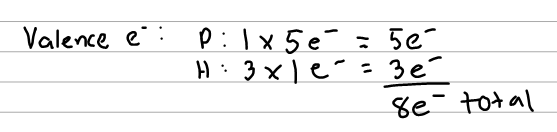# Problem: Draw the Lewis structure of PH 3.

###### FREE Expert Solution
94% (133 ratings)
###### FREE Expert Solution

The questions ask us to draw the Lewis structure so the first thing we need to know is the number of valence electrons. We know that P has 5 e- since it is from group 5a and H will have 1 e- each.Next, we draw the atoms. P will be the central atom here since H never goes in the center. Then we form bonds with each hydrogen.

94% (133 ratings)###### Problem Details

Draw the Lewis structure of PH 3.

What scientific concept do you need to know in order to solve this problem?

Our tutors have indicated that to solve this problem you will need to apply the Lewis Dot Structure concept. You can view video lessons to learn Lewis Dot Structure. Or if you need more Lewis Dot Structure practice, you can also practice Lewis Dot Structure practice problems.

What is the difficulty of this problem?

Our tutors rated the difficulty ofDraw the Lewis structure of PH 3....as low difficulty.

How long does this problem take to solve?

Our expert Chemistry tutor, Dasha took undefined 52 seconds to solve this problem. You can follow their steps in the video explanation above.

What professor is this problem relevant for?

Based on our data, we think this problem is relevant for Professor Henricks' class at KSU.